Note

# Historic comparison PV and wind (Germany)¶

In this example we are examining the ranalysed power feed-ins calculated by atlite. Based on data for capacity distributions and weather during the year 2013, we try to match historical statistics.

## Importing packages¶

:

import atlite
import xarray as xr
import pandas as pd
import scipy.sparse as sp
import numpy as np

import pgeocode
from collections import OrderedDict

:

import matplotlib.pyplot as plt
%matplotlib inline

import seaborn as sns
sns.set_style('whitegrid')


1. We need to download the locations of all the PV installations in Germany to later tell atlite where to setup the PV panels and with which capacity. This information is available in Germany (thanks to the EEG feed-in-tariffs in the so-called “Anlagenregister”).

2. We also download a reference time-series to compare our results against later. We retrieve the data from https://open-power-system-data.org which in return gets it from ENTSO-E.

3. Finally we also download a cutout of weather data from the ERA5 dataset containing Germany and the year we want to examine (2012).

:

import requests
import os
import zipfile

# variant of http://stackoverflow.com/a/16696317
if not os.path.exists(local_filename):
r = requests.get(url, stream=True)
with open(local_filename, 'wb') as f:
for chunk in r.iter_content(chunk_size=1024):
if chunk:
f.write(chunk)
return local_filename


## Reference time-series¶

The reference is downloaded from Open Power System Data (OPSD) and contains data reported by the TSOs and DSOs.

:

opsd_fn = download_file('https://data.open-power-system-data.org/index.php?package=time_series&version=2019-06-05&action=customDownload&resource=3&filter%5B_contentfilter_cet_cest_timestamp%5D%5Bfrom%5D=2012-01-01&filter%5B_contentfilter_cet_cest_timestamp%5D%5Bto%5D=2013-05-01&filter%5BRegion%5D%5B%5D=DE&filter%5BVariable%5D%5B%5D=solar_generation_actual&filter%5BVariable%5D%5B%5D=wind_generation_actual&downloadCSV=Download+CSV',
'time_series_60min_singleindex_filtered.csv')

:

opsd = pd.read_csv(opsd_fn, parse_dates=True, index_col=0)

# we later use the (in current version) timezone unaware datetime64
# to work together with this format, we have to remove the timezone
# timezone information. We are working with UTC everywhere.

opsd.index = opsd.index.tz_convert(None)

# We are only interested in the 2012 data
opsd = opsd[("2011" < opsd.index) & (opsd.index < "2013")]


## PV locations (“Anlagenregister”)¶

Download and unzip the archive containing all reported PV installations in Germany in 2011 from energymap.info.

:

eeg_fn = download_file('http://www.energymap.info/download/eeg_anlagenregister_2015.08.utf8.csv.zip',
'eeg_anlagenregister_2015.08.utf8.csv.zip')

with zipfile.ZipFile(eeg_fn, "r") as zip_ref:
zip_ref.extract("eeg_anlagenregister_2015.08.utf8.csv")


# Create a Cutout from ERA5¶

:

import cartopy.io.shapereader as shpreader
import geopandas as gpd
de_record = list(filter(lambda c: c.attributes['ISO_A2'] == 'DE', shp.records()))
de = gpd.GeoSeries({**de_record.attributes, 'geometry':de_record.geometry})
x1, y1, x2, y2 = de['geometry'].bounds

/home/fabian/.miniconda3/lib/python3.7/site-packages/ipykernel_launcher.py:5: FutureWarning:     You are passing non-geometry data to the GeoSeries constructor. Currently,
it falls back to returning a pandas Series. But in the future, we will start
"""

:

cutout = atlite.Cutout('germany-2012',
module='era5',
x=slice(x1-.2,x2+.2), y=slice(y1-.2, y2+.2),
chunks={'time':100},
time="2012")

/home/fabian/vres/py/atlite/atlite/cutout.py:160: UserWarning: Arguments module, x, y, time are ignored, since cutout is already built.
warn(f'Arguments {", ".join(cutoutparams)} are ignored, since '

:

cutout.prepare()

:

<Cutout "germany-2012">
x = 5.75 ⟷ 15.00, dx = 0.25
y = 47.25 ⟷ 55.25, dy = 0.25
time = 2012-01-01 ⟷ 2012-12-31, dt = H
module = era5
prepared_features = ['height', 'wind', 'influx', 'temperature', 'runoff']


Downloading the cutout can take a few seconds or even an hour, depending on your internet connection and whether the dataset was recently requested from the data set provider (and is thus cached on their premise). For us this took ~2 minutes the first time. Preparing it again (a second time) is snappy (for whatever reason you would want to download the same cutout twice).

# Creating time-series¶

## Generate capacity layout¶

The capacity layout represents the installed generation capacities in MW in each of the cutout’s grid cells. For this example we have generation capacities in kW on a postal code (and partially even more detailed) level. Using the function below, we load the data, fill in geocoordinates where missing for all capacities and then dissolve them to the grid raster provided by the cutout. The dissolving is done by aggregating the capacities to their respective closest grid cell center obtained from the cutout.grid_coordinates().

:

def capacity_layout(cutout, typ, cap_range=None, until=None):
"""Aggregate selected capacities to the cutouts grid into a capacity layout.

Parameters
----------
cutout : atlite.cutout
The cutout for which the capacity layout is contructed.
typ : str
Type of energy source, e.g. "Solarstrom" (PV), "Windenergie" (wind).
cap_range : (optional) list-like
Two entries, limiting the lower and upper range of capacities (in kW)
to include. Left-inclusive, right-exclusive.
until : str
String representation of a datetime object understood by pandas.to_datetime()
for limiting to installations existing until this datetime.

"""

# Load locations of installed capacities and remove incomplete entries
cols = OrderedDict((('installation_date', 0),
('plz', 2), ('city', 3),
('type', 6),
('capacity', 8), ('level', 9),
('lat', 19), ('lon', 20),
('validation', 22)))
sep=';', decimal=',', thousands='.',
usecols=list(cols.values()),
names=list(cols.keys()),
# German postal codes can start with '0' so we need to treat them as str
dtype={'plz':str},
parse_dates=['installation_date'],
na_values=('O04WF', 'keine'))

database = database[(database['validation'] == 'OK') & (database['plz'].notna())]

# Query postal codes <-> coordinates mapping
de_nomi = pgeocode.Nominatim('de')
plz_coords = de_nomi.query_postal_code(database['plz'].unique())
plz_coords = plz_coords.set_index('postal_code')

# Fill missing lat / lon using postal codes entries
database.loc[database['lat'].isna(), 'lat'] = database['plz'].map(plz_coords['latitude'])
database.loc[database['lon'].isna(), 'lon'] = database['plz'].map(plz_coords['longitude'])

# Ignore all locations which have not be determined yet
database = database[database['lat'].notna() & database['lon'].notna()]

# Select data based on type (i.e. solar/PV, wind, ...)
data = database[database['type'] == typ].copy()

# Optional: Select based on installation day
if until is not None:
data = data[data['installation_date'] < pd.to_datetime(until)]

# Optional: Only installations within this caprange (left inclusive, right exclusive)
if cap_range is not None:
data = data[(cap_range <= data['capacity']) & (data['capacity'] < cap_range)]

# Determine nearest cells from cutout
cells = gpd.GeoDataFrame({'geometry': cutout.grid_cells,
'lon': cutout.grid_coordinates()[:,0],
'lat': cutout.grid_coordinates()[:,1]})

nearest_cell = cutout.data.sel({'x': data.lon.values,
'y': data.lat.values},
'nearest').coords

# Map capacities to closest cell coordinate
data['lon'] = nearest_cell.get('lon').values
data['lat'] = nearest_cell.get('lat').values

new_data = data.merge(cells, how='inner')

# Sum capacities for each grid cell (lat, lon)
# then: restore lat lon as coumns
# then: rename and reindex to match cutout coordinates
new_data = new_data.groupby(['lat','lon']).sum()

layout = new_data.reset_index().rename(columns={'lat':'y','lon':'x'})\
.set_index(['y','x']).capacity\
.to_xarray().reindex_like(cutout.data)

layout = (layout/1e3).fillna(.0).rename('Installed Capacity [MW]')

return layout


### Examine Solar Feed-Ins¶

The layout defines the production capacity per grid cell. Let’s see how it looked like in 2013.

:

solar_layout = capacity_layout(cutout, 'Solarstrom', until="2012")

solar_layout.plot(cmap="inferno_r", size=8, aspect=1)
plt.title("Installed PV in Germany until 2012")
plt.tight_layout()

The order of elements returned by grid_coordinates changed. Check the output of your workflow for correctness.
The order of elements returned by grid_coordinates changed. Check the output of your workflow for correctness.
/home/fabian/.miniconda3/lib/python3.7/site-packages/xarray/core/indexing.py:1375: PerformanceWarning: Slicing is producing a large chunk. To accept the large
chunk and silence this warning, set the option
...     array[indexer]

To avoid creating the large chunks, set the option
...     array[indexer]
value = value[(slice(None),) * axis + (subkey,)]What did the total production of this capacity distribution looked like? We pass the layout to the conversion funtion cutout.pv. This calculates the total production over the year.

:

pv = cutout.pv(panel="CSi", orientation={'slope': 30., 'azimuth': 0.}, layout=solar_layout)

[########################################] | 100% Completed |  2.7s


As OPSD also provides data on the total production, let’s compare those two.

:

pv.squeeze().to_series()

:

time
2012-01-01 00:00:00    0.0
2012-01-01 01:00:00    0.0
2012-01-01 02:00:00    0.0
2012-01-01 03:00:00    0.0
2012-01-01 04:00:00    0.0
...
2012-12-31 19:00:00    0.0
2012-12-31 20:00:00    0.0
2012-12-31 21:00:00    0.0
2012-12-31 22:00:00    0.0
2012-12-31 23:00:00    0.0
Length: 8784, dtype: float64

:

compare = pd.DataFrame(dict(atlite=pv.squeeze().to_series(), opsd=opsd['DE_solar_generation_actual'])) /1e3 # in GW
compare.resample('1W').mean().plot(figsize=(8,5))
plt.ylabel("Feed-In [GW]")
plt.title('PV time-series Germany 2012')
plt.tight_layout()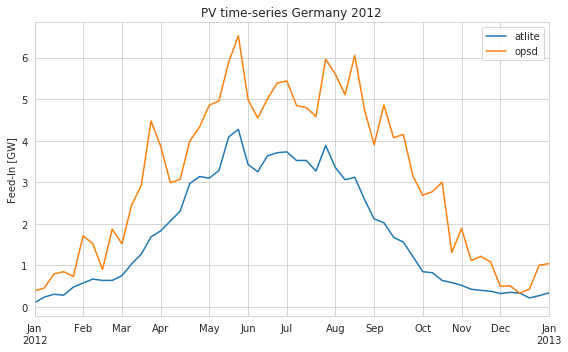Looks like atlite is underestimating the power feed-ins. This might be caused by conservative technological assumptions (slope, panel).

For example when we set an optimal slope of the panels. The production per week looks much better

:

pv_opt = cutout.pv(panel="CSi", orientation='latitude_optimal', layout=solar_layout)
compare_opt = pd.DataFrame(dict(atlite=pv_opt.squeeze().to_series(), opsd=opsd['DE_solar_generation_actual']))/1e3 # in GW
compare_opt.resample('1W').mean().plot(figsize=(8,5))
plt.ylabel("Feed-In [GW]")
plt.title('PV time-series Germany 2012')
plt.tight_layout()

[########################################] | 100% Completed |  2.8s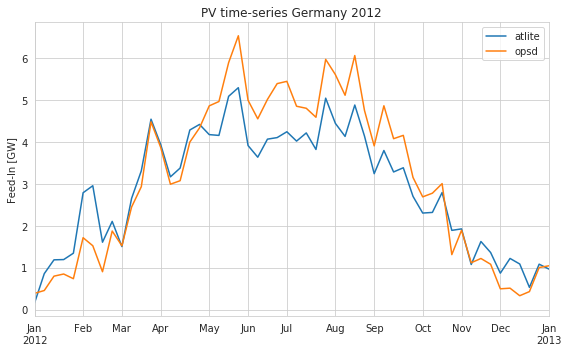Looks better :) How about zooming in? Let’s plot a specific week. We see that the peaks are differing a bit. In this case atlite alternates between over and underestimating a bit.

But for a reanalysis, not too bad…

:

compare_opt.loc["2012-04"].plot(figsize=(10,6))
plt.ylabel('Feed-in [GW]')
plt.title('PV time-series Germany April 2012')
plt.tight_layout()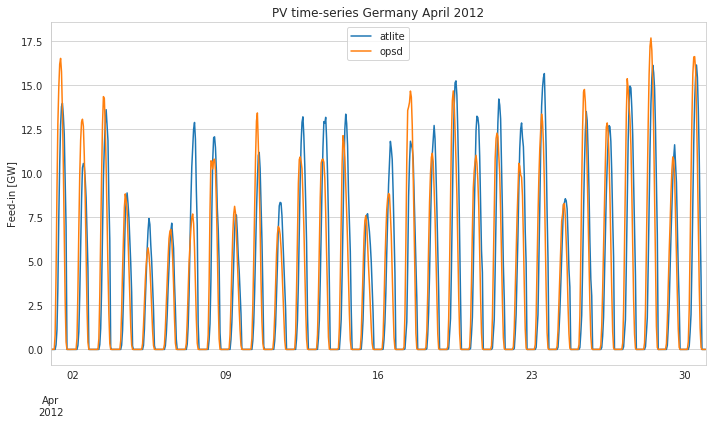:

fig, (ax1, ax2) = plt.subplots(1,2, subplot_kw={'aspect': 'equal', 'xlim':[0,20]}, figsize=(12,16), sharey=True)
compare.plot(x='atlite', y='opsd', kind='scatter', s=1, ax=ax1, title='Slope 30°')
compare_opt.plot(x='atlite', y='opsd', kind='scatter', s=1, ax=ax2, title='Slope Optimal')

ax1.plot([0,20],[0,20], c='gray')
ax2.plot([0,20],[0,20], c='gray')
plt.tight_layout()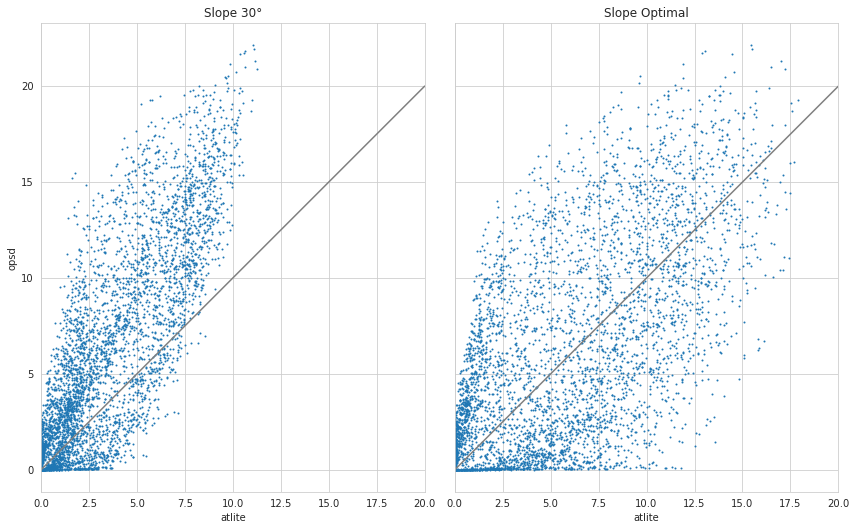Whereas in the spatial aggregation the optimal slope performs better, on a detailed level the spread is very high. Thus the steady slope of 30° might be a saver choice knowing that this tends to underestimate.

Let’s look at the duration curves of the 30° slope pv timeseries.

:

compare['atlite'].sort_values(ascending=False).reset_index(drop=True).plot(figsize=(10,6))
compare['opsd'].sort_values(ascending=False).reset_index(drop=True).plot(figsize=(10,6))
plt.legend()
plt.title('Duration curves')
plt.tight_layout()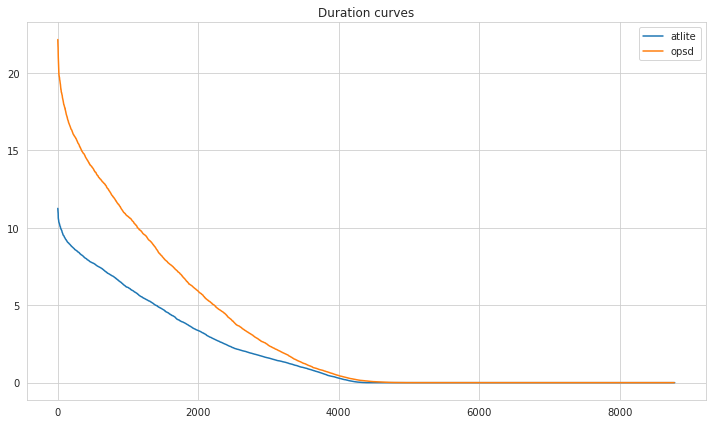### Examine Wind Feed-Ins¶

Now we want to examine the wind potentials in Germany for year 2013.

These wind turbines are available in atlite.

:

for t in atlite.windturbines: print(f'* {t}')

* Vestas_V47_660kW
* Enercon_E82_3000kW
* Siemens_SWT_2300kW
* Suzlon_S82_1.5_MW
* Vestas_V112_3MW
* NREL_ReferenceTurbine_5MW_offshore
* Vestas_V164_7MW_offshore
* Enercon_E101_3000kW
* Vestas_V90_3MW
* Siemens_SWT_107_3600kW
* Vestas_V80_2MW_gridstreamer
* Bonus_B1000_1000kW
* Vestas_V112_3MW_offshore
* Vestas_V25_200kW
* Enercon_E126_7500kW
* Vestas_V66_1750kW


We define capacity range to roughly match the wind turbine type.

:

turbine_categories = [
dict(name='Vestas_V25_200kW', up=400.),
dict(name='Vestas_V47_660kW', up=700.),
dict(name='Bonus_B1000_1000kW', up=1100.),
dict(name='Suzlon_S82_1.5_MW', up=1600.),
dict(name='Vestas_V66_1750kW', up=1900.),
dict(name='Vestas_V80_2MW_gridstreamer', up=2200.),
dict(name='Siemens_SWT_2300kW', up=2500.),
dict(name='Vestas_V90_3MW', up=50000.)
]


:

low = 0
for index, turbine_cat in enumerate(turbine_categories):

layout = capacity_layout(cutout, 'Windkraft',
cap_range=[low,turbine_cat['up']],
until="2012")

turbine_categories[index]['layout'] = layout
low = turbine_cat['up']

The order of elements returned by grid_coordinates changed. Check the output of your workflow for correctness.
The order of elements returned by grid_coordinates changed. Check the output of your workflow for correctness.
/home/fabian/.miniconda3/lib/python3.7/site-packages/xarray/core/indexing.py:1375: PerformanceWarning: Slicing is producing a large chunk. To accept the large
chunk and silence this warning, set the option
...     array[indexer]

To avoid creating the large chunks, set the option
...     array[indexer]
value = value[(slice(None),) * axis + (subkey,)]
The order of elements returned by grid_coordinates changed. Check the output of your workflow for correctness.
The order of elements returned by grid_coordinates changed. Check the output of your workflow for correctness.
/home/fabian/.miniconda3/lib/python3.7/site-packages/xarray/core/indexing.py:1375: PerformanceWarning: Slicing is producing a large chunk. To accept the large
chunk and silence this warning, set the option
...     array[indexer]

To avoid creating the large chunks, set the option
...     array[indexer]
value = value[(slice(None),) * axis + (subkey,)]
The order of elements returned by grid_coordinates changed. Check the output of your workflow for correctness.
The order of elements returned by grid_coordinates changed. Check the output of your workflow for correctness.
/home/fabian/.miniconda3/lib/python3.7/site-packages/xarray/core/indexing.py:1375: PerformanceWarning: Slicing is producing a large chunk. To accept the large
chunk and silence this warning, set the option
...     array[indexer]

To avoid creating the large chunks, set the option
...     array[indexer]
value = value[(slice(None),) * axis + (subkey,)]
The order of elements returned by grid_coordinates changed. Check the output of your workflow for correctness.
The order of elements returned by grid_coordinates changed. Check the output of your workflow for correctness.
/home/fabian/.miniconda3/lib/python3.7/site-packages/xarray/core/indexing.py:1375: PerformanceWarning: Slicing is producing a large chunk. To accept the large
chunk and silence this warning, set the option
...     array[indexer]

To avoid creating the large chunks, set the option
...     array[indexer]
value = value[(slice(None),) * axis + (subkey,)]
The order of elements returned by grid_coordinates changed. Check the output of your workflow for correctness.
The order of elements returned by grid_coordinates changed. Check the output of your workflow for correctness.
/home/fabian/.miniconda3/lib/python3.7/site-packages/xarray/core/indexing.py:1375: PerformanceWarning: Slicing is producing a large chunk. To accept the large
chunk and silence this warning, set the option
...     array[indexer]

To avoid creating the large chunks, set the option
...     array[indexer]
value = value[(slice(None),) * axis + (subkey,)]
The order of elements returned by grid_coordinates changed. Check the output of your workflow for correctness.
The order of elements returned by grid_coordinates changed. Check the output of your workflow for correctness.
/home/fabian/.miniconda3/lib/python3.7/site-packages/xarray/core/indexing.py:1375: PerformanceWarning: Slicing is producing a large chunk. To accept the large
chunk and silence this warning, set the option
...     array[indexer]

To avoid creating the large chunks, set the option
...     array[indexer]
value = value[(slice(None),) * axis + (subkey,)]
The order of elements returned by grid_coordinates changed. Check the output of your workflow for correctness.
The order of elements returned by grid_coordinates changed. Check the output of your workflow for correctness.
/home/fabian/.miniconda3/lib/python3.7/site-packages/xarray/core/indexing.py:1375: PerformanceWarning: Slicing is producing a large chunk. To accept the large
chunk and silence this warning, set the option
...     array[indexer]

To avoid creating the large chunks, set the option
...     array[indexer]
value = value[(slice(None),) * axis + (subkey,)]
The order of elements returned by grid_coordinates changed. Check the output of your workflow for correctness.
The order of elements returned by grid_coordinates changed. Check the output of your workflow for correctness.
/home/fabian/.miniconda3/lib/python3.7/site-packages/xarray/core/indexing.py:1375: PerformanceWarning: Slicing is producing a large chunk. To accept the large
chunk and silence this warning, set the option
...     array[indexer]

To avoid creating the large chunks, set the option
...     array[indexer]
value = value[(slice(None),) * axis + (subkey,)]


We create a layout for each capacity range, each with a different windturbine model

:

wind = xr.Dataset()
for turbine_cat in turbine_categories:
name = f"< {turbine_cat['up']} kW"
wind[name] = cutout.wind(turbine=turbine_cat['name'], layout=turbine_cat['layout'], show_progress=False)

wind['total'] = sum(wind[c] for c in wind)
wind

:

<xarray.Dataset>
Dimensions:       (dim_0: 1, time: 8784)
Coordinates:
* time          (time) datetime64[ns] 2012-01-01 ... 2012-12-31T23:00:00
* dim_0         (dim_0) int64 0
Data variables:
< 400.0 kW    (time, dim_0) float64 14.37 17.37 21.26 ... 76.24 74.27 72.48
< 700.0 kW    (time, dim_0) float64 260.0 291.3 344.2 ... 1.276e+03 1.27e+03
< 1100.0 kW   (time, dim_0) float64 175.9 191.8 213.9 ... 846.2 829.2 821.8
< 1600.0 kW   (time, dim_0) float64 1.125e+03 1.209e+03 ... 4.563e+03
< 1900.0 kW   (time, dim_0) float64 322.0 356.3 ... 1.738e+03 1.729e+03
< 2200.0 kW   (time, dim_0) float64 991.7 1.05e+03 ... 4.803e+03 4.818e+03
< 2500.0 kW   (time, dim_0) float64 545.8 599.2 703.2 ... 1.897e+03 1.89e+03
< 50000.0 kW  (time, dim_0) float64 269.6 298.4 ... 1.023e+03 1.006e+03
total         (time, dim_0) float64 3.704e+03 4.013e+03 ... 1.617e+04

Again, let’s compare the result with the feed-in statistics from OPSD. We add a extra column for wind turbines with capacity lower than 1600 kW

:

compare = pd.DataFrame({"atlite":wind['total'].squeeze().to_series(),
"< 1600 kW":wind['< 1600.0 kW'].squeeze().to_series(),
"opsd":opsd['DE_wind_generation_actual']})

compare = compare/1e3 # in GW

:

compare.resample('1W').mean().plot(figsize=(10,6))
plt.ylabel('Feed-in [GW]')
plt.title('Wind time-series Germany 2012')
plt.tight_layout()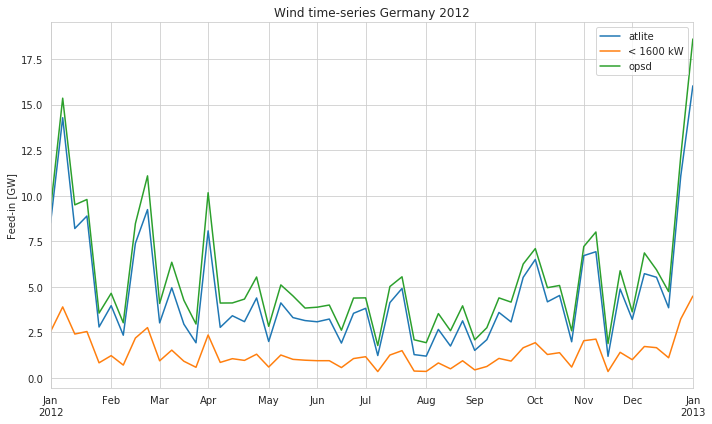:

compare.loc["2012-04"].plot(figsize=(10,6))
plt.ylabel('Feed-in [GW]')
plt.title('Wind time-series Germany April 2012')
plt.tight_layout()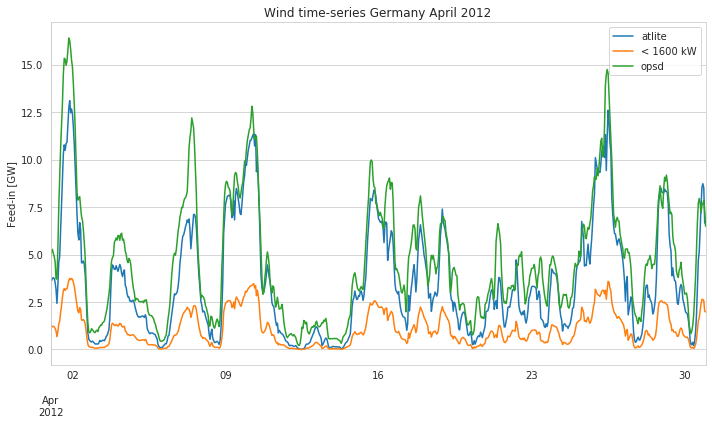:

ax = compare.plot(x='atlite', y='opsd', kind='scatter', figsize=(12,8))
ax.set_aspect('equal')
ax.set_xlim(0,30)
ax.plot([0,30],[0,30],c='gray')
plt.tight_layout()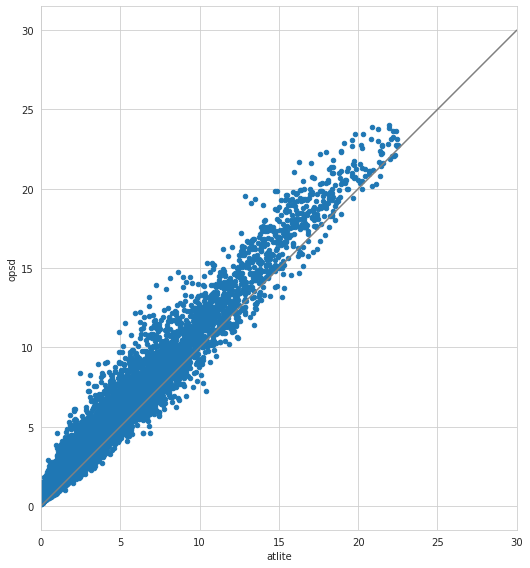:

compare['atlite'].sort_values(ascending=False).reset_index(drop=True).plot(figsize=(10,6))
compare['opsd'].sort_values(ascending=False).reset_index(drop=True).plot(figsize=(10,6))
plt.legend()
plt.title('Duration curves')
plt.ylabel('Wind Feed-in [GW]')
plt.xlabel('Accumulated hours')
plt.tight_layout()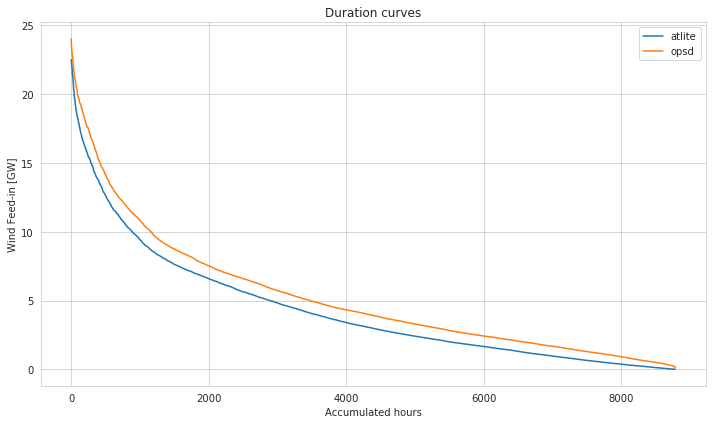Looks quite aggreeable!

### Splitting time-series into shapes¶

The generation time-series can also be aggregated based on shapes. In this example, we aggregate on the basis of the German “Laender” (federal states).

:

shp = shpreader.Reader(shpreader.natural_earth(resolution='10m',
category='cultural',
de_records = list(filter(lambda r: r.attributes['iso_3166_2'].startswith('DE'), shp.records()))
laender = gpd.GeoDataFrame([{**r.attributes, 'geometry':r.geometry} for r in de_records])\
.rename(columns={"iso_3166_2":"state"}).set_index("state")

:

x1, y1, x2, y2 = de['geometry'].bounds

:

print(type(laender.loc['DE-TH'].geometry))
laender.loc['DE-TH']

<class 'shapely.geometry.polygon.Polygon'>

:

featurecla                                   Admin-1 scale rank
scalerank                                                     3
diss_me                                                    1577
wikipedia                                                  None
...
name_tr                                              TÃ¼ringiya
name_vi                                              ThÃ¼ringen
name_zh                                               å¾ææ ¹
ne_id                                                1159310983
geometry      POLYGON ((9.949863314740185 51.30301870404992,...
Name: DE-TH, Length: 83, dtype: object

:

pv = cutout.pv(panel="CSi", orientation={'slope': 30., 'azimuth': 0.},
shapes=laender['geometry'],
layout=solar_layout)

[########################################] | 100% Completed |  2.8s

:

pv.sel(state=['DE-TH', 'DE-BY']).to_pandas().resample('1W').mean().plot(figsize=(10,6))

:

<matplotlib.axes._subplots.AxesSubplot at 0x7f1e7a94d7d0>